# Visualizing the concentric hulls of E8 with the 24-cells of H4 & H4Φ

I’ve had a number of related posts on this topic, but I wanted to present a few new pictures and PDF documents that combine the 7 concentric hulls forming Platonic solid related sets of E8 vertices projected to 3D with vertices of five pairs of 24-cell objects.

These break down the E8 structure into familiar 3D objects, such as the icosahedron with its dual the dodecahedron, the icosidodecahdron, and the 16-cell with its dual the 8-cell (aka. Tesseract) combined to create the self-dual 24-cell.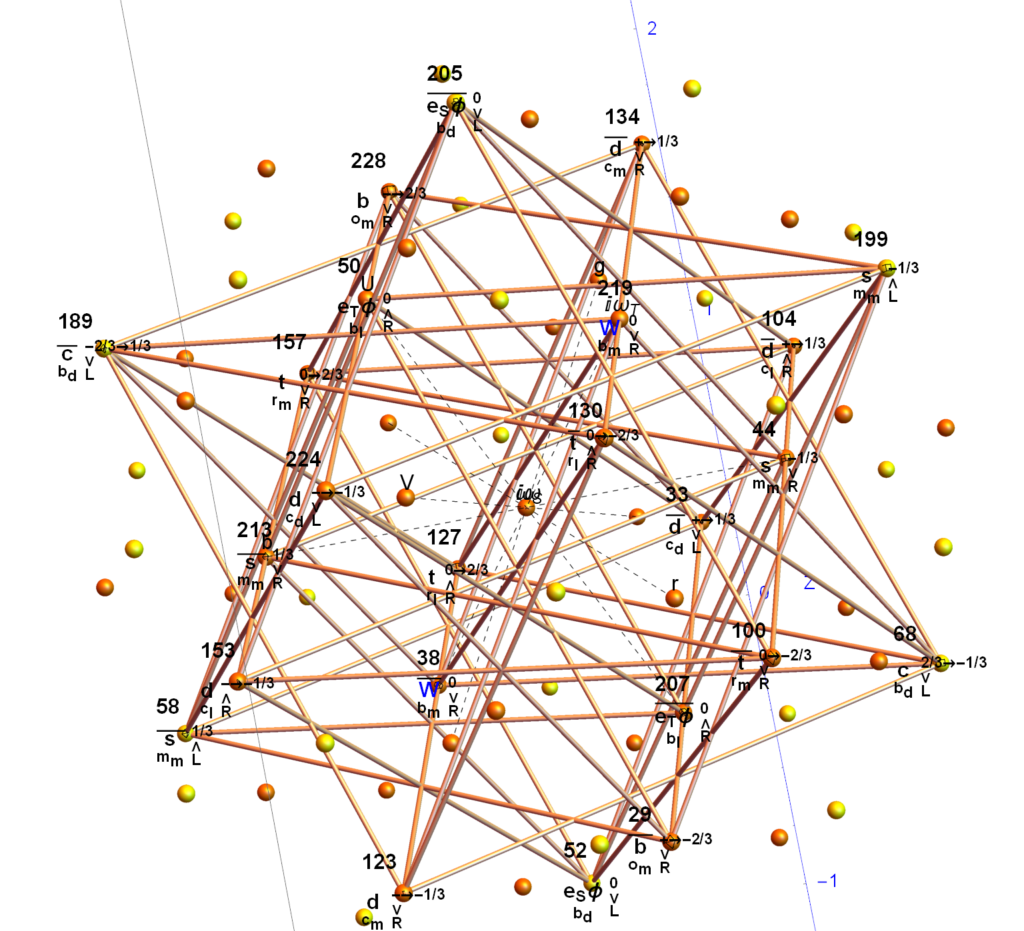The yellow spheres are those of the outer hull of singular 30 vertices of an icosidodecahedron. The orange spheres are pairs of icosahedron or dodecahedron vertices. The edges with numbered vertices form one of five 24-cells in the 120 vertex H4Φ scaled 600-cell within E8. Specifically, it is the 3rd rotation (of 4 π/5 rotations) within the 96 vertices of the snub-24-cell. You can see that 6 of the yellow vertices connect to the 24-cell. Rotating that 24-cell four times in 3-space by π/5 gives the connections to the rest of the vertices in H4Φ and completes the 30 vertex icosidodecahedron . The same is true for the 120 vertices of H4 using the corresponding 24-cell in H4.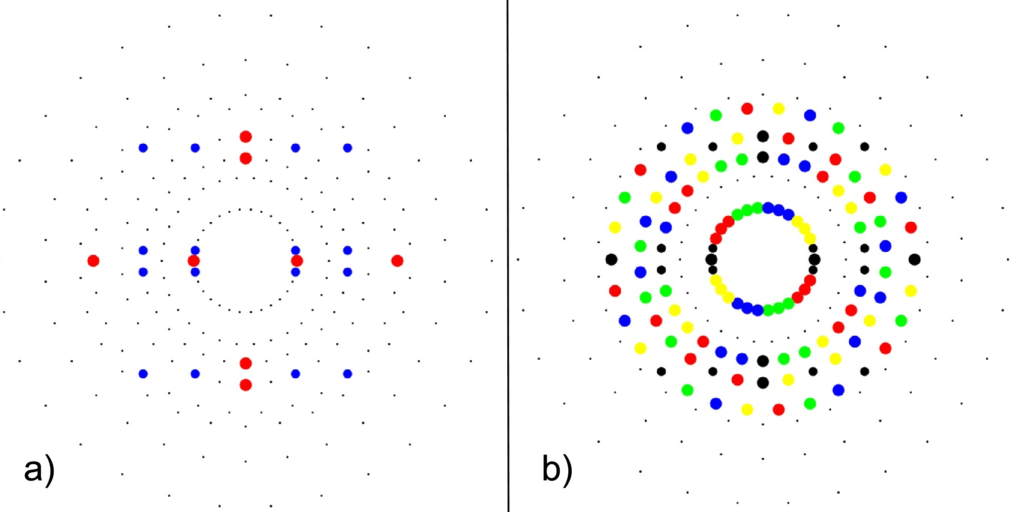a) 24-cell highlighting the 16-cell (red on-axis vertices) and 8-cell (blue off-axis vertices)b) Snub 24-cell highlighting four π/5 rotations of the 24-cell (black) in red, green, blue, yellow.

The following paper hulls-24cells-combined.pdf (13MB) & interactive Mathematica Notebook hulls-24cells-combined.nb (34MB) contains a comprehensive set of images that show the contents of the 7 concentric hulls of Platonic solid related shapes as well as their integration with one of the rotations of 24-cells in H4 and H4Φ in E8 (the same one used in the image above).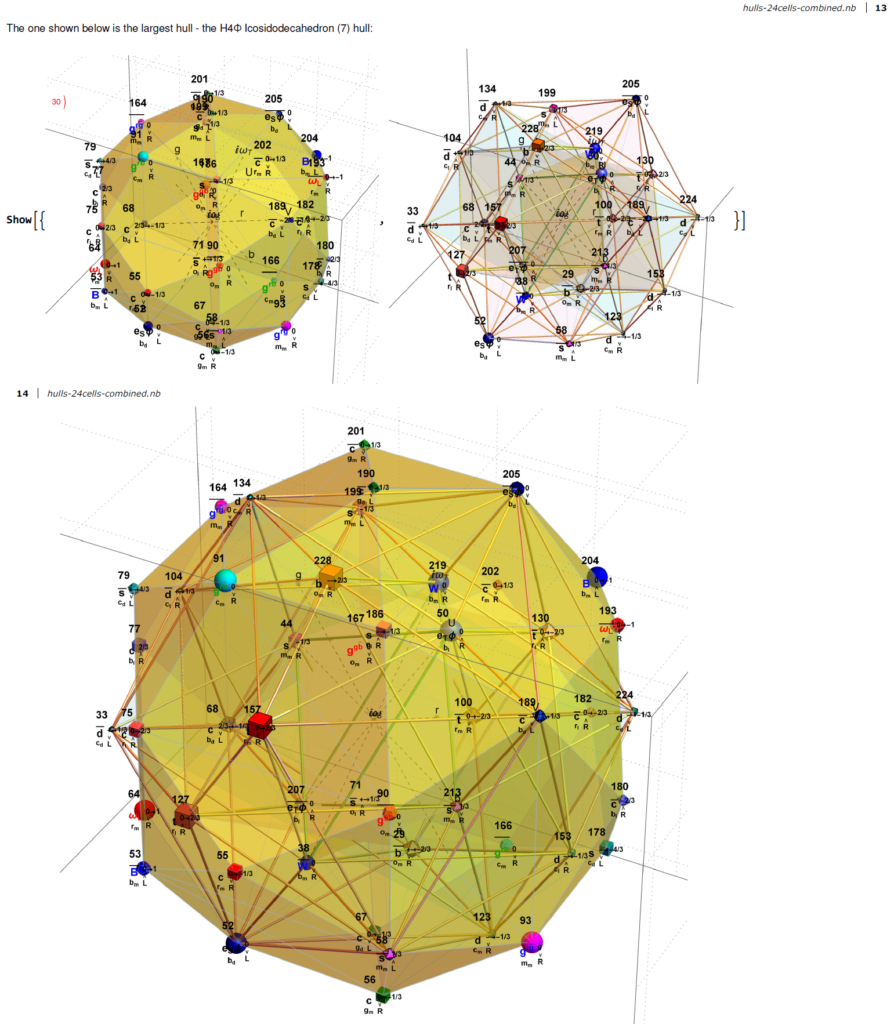Snapshot from the paper showing the 3rd rotation of the snub 24-cell and the icosidodecahedron of H4Φ

In the E8 Petrie projection, every hull (each with 2 or 4 overlapping vertices) pair into left/right patterns. See the set of four icosahedrons that occupy the 3rd hull in both 2D and 3D (the yellow edge sets belongs to the H4Φ and the blue sets belong to H4) :

The pair of dodecahedrons that occupy the 5th hull in both 2D and 3D (the yellow edge set belongs to the H4Φ and the blue belong to H4) :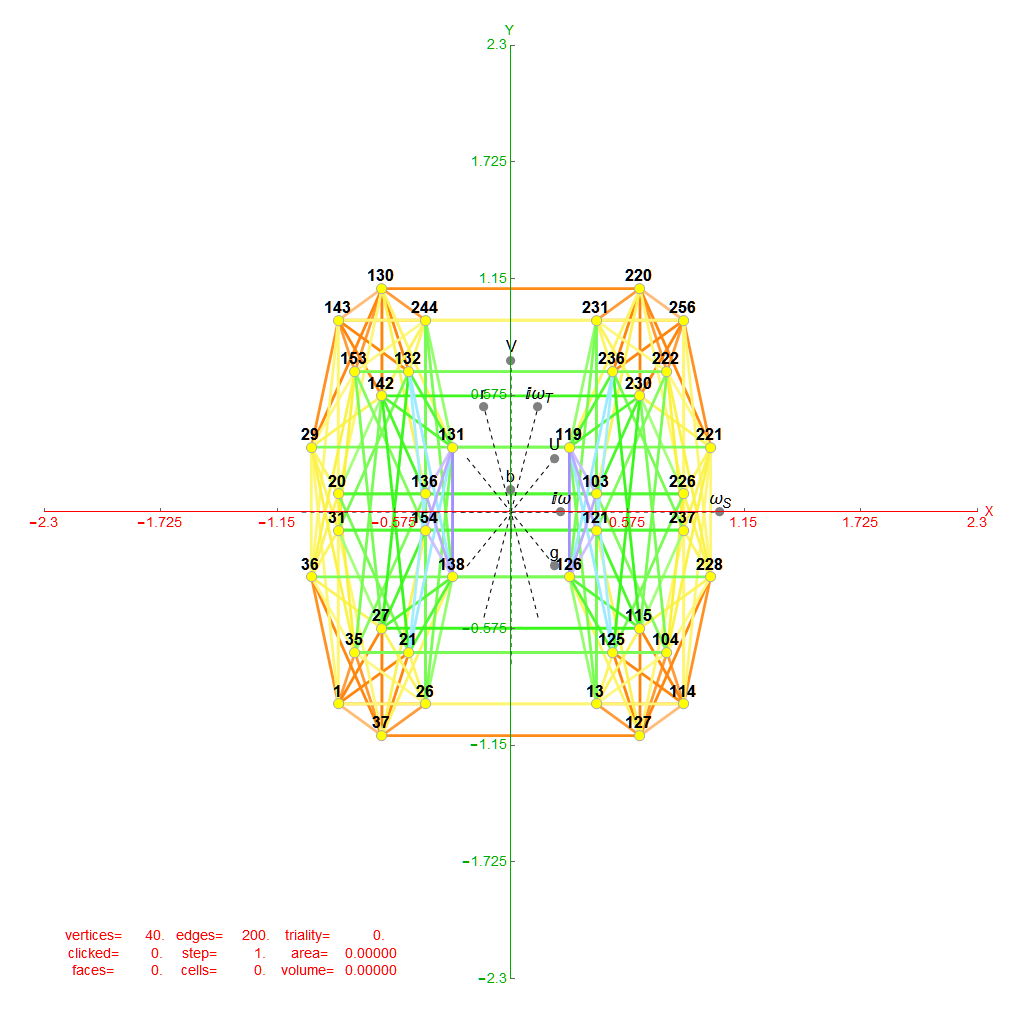5th hull Dodecahedrons in 2D Petrie Projection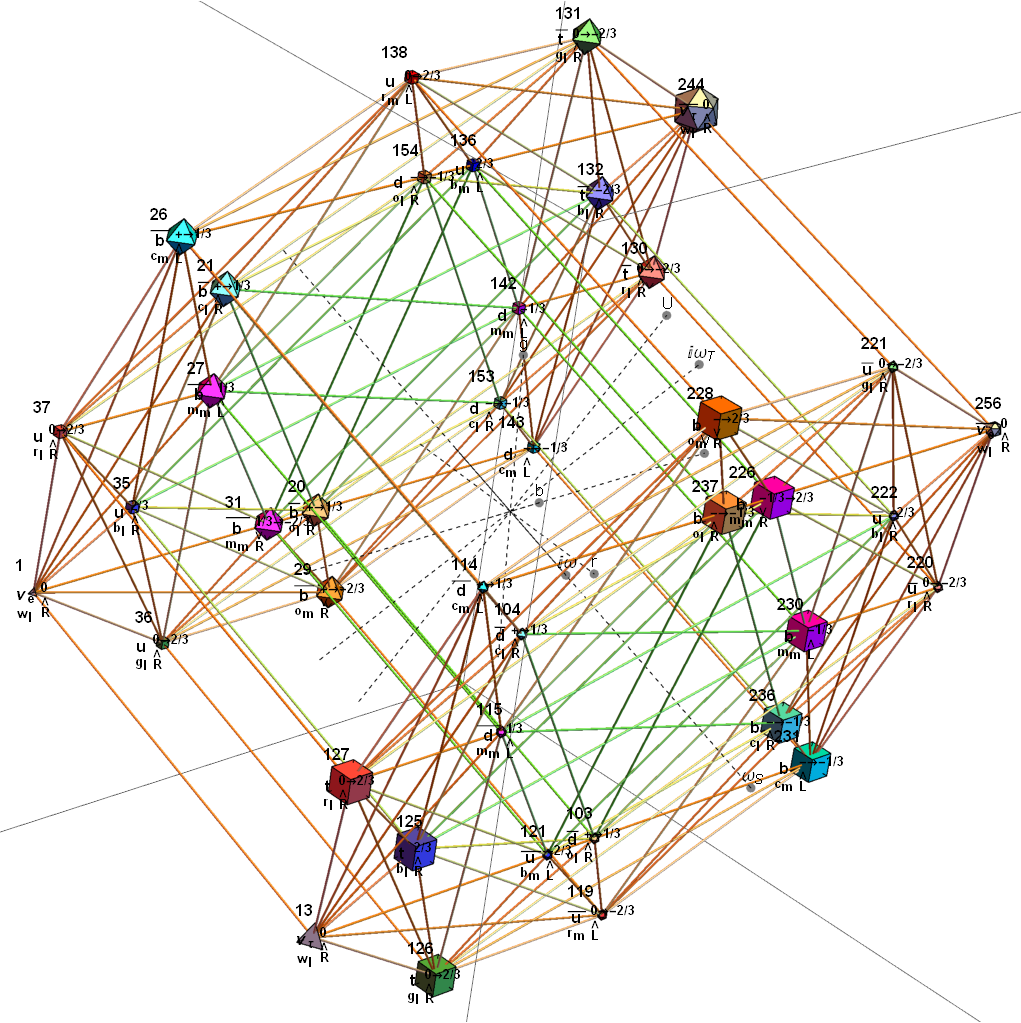5th hull Dodecahedrons in 3D Petrie Projection (also showing the physics particle assignment)

Below is an animation that cycles through the sequence of 2D Petrie Projections of the pairs of hulls. One cycle shows each frame individually and the other builds the E8 Petrie from the previous 2D hull.

Below are images of the left (H4) and right (H4Φ) 2D Petrie projections of the hulls (which are defined by the Norm’s of 3D projected vertices using the E8->H4 folding matrix rows 2-4 as basis vectors). The two 30 vertex 4 & 7 Icosidodecahedron hulls and four 0th hull (points) are omitted leaving the gaps in the diagram. When these gap vertices are combined with the 48 3rd hull Icosahedrons (above), they make up the 112 integer D8 group assigned to the Bosons (48) and 2nd generation Fermions (64) in the physics model described below.

The combined set of hulls projected using the rows of the folding matrix as basis vectors is shown below from previous work: